# K means Clustering

This is a first type of algorithm in unsupervised data.

This is similar to KNN which assumes that similar observations are grouped together are distance- based algorithms with only difference being that in KNN we check that labels of K nearest neighbors and then decide corresponding label of our point while in K means we find that why K nearest neighbors are similar to the point in consideration…

Steps for Algorithm

1. Specify the desired number of clusters K
2. Randomly assign each data point to a cluster
3. Compute cluster centroids(mean of all points within the cluster)
4. Re-assign each point to the closest cluster centroid
5. Re-compute cluster centroids
6. Repeat steps 4 and 5 until no improvements are possible : Similarly, we’ll repeat the 4th and 5th steps until we’ll reach global optima. When there will be no further switching of data points between two clusters for two successive repeats or the centroid stops moving and becomes static, it will mark the termination of the algorithm if not explicitly mentioned.

Ways to determine K

Although no one can pick this with certainty unless it is directed to analyst by stakeholders, we still methods directing to the best to use k.

These methods include direct methods and statistical testing methods:

1. Direct methods: consists of optimizing a criterion, such as the within cluster sums of squares or the average silhouette. The corresponding methods are named elbow and silhouette methods, respectively.
2. Statistical testing methods: consists of comparing evidence against null hypothesis. An example is the gap statistic.

Elbow Method

1. Compute clustering algorithm (e.g., k-means clustering) for different values of k. For instance, by varying k from 1 to 10 clusters.
2. For each k, calculate the total within-cluster sum of square (wss).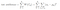where mu_k is centroid of each cluster k
1. Plot the curve of wss according to the number of clusters k.
2. The location of a bend (knee) in the plot is generally considered as an indicator of the appropriate number of clusters.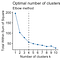This suggests K=4

Average Silhouette method

1. Compute clustering algorithm (e.g., k-means clustering) for different values of k. For instance, by varying k from 1 to 10 clusters.
2. For each k, calculate the average silhouette of observations (avg.sil).
3. Plot the curve of avg.sil according to the number of clusters k.
4. The location of the maximum is considered as the appropriate number of clusters.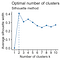This suggests K=2

Gap Statistics Method

1. Cluster the observed data, varying the number of clusters from k = 1, …, kmax, and compute the corresponding total within intra-cluster variation Wk.
2. Generate B reference data sets with a random uniform distribution. Cluster each of these reference data sets with varying number of clusters k = 1, …, kmax, and compute the corresponding total within intra-cluster variation Wkb.
3. Compute the estimated gap statistic as the deviation of the observed Wk value from its expected value Wkb under the null hypothesis:

Compute also the standard deviation of the statistics.

4. Choose the number of clusters as the smallest value of k such that the gap statistic is within one standard deviation of the gap at k+1: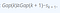s is std of gap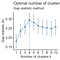This suggests K=4

Points to note:

1. Now if you want to visualize the clusters, it is only possible when you select any 2 independent variables out of your multiple variables present, to do so try performing PCA (Principal Component analysis) which will give you PC1, AND PC2 as axis in visualizing. This is so because first two principal components will cover large amount of variation present in your data.
2. K means is very sensitive to outliers. (PAM is less sensitive to outliers which uses optimal average silhouette width)
3. Although it can easily be run on large datasets, it is sensitive to initial random selection of cluster centers. Similarly it varies with ordering of data, since initializing is random. (Try reordering and validating the results)

Resources:

Problem Solver, Data Science, Actuarial Science, Knowledge Sharer, Hardcore Googler

## More from Shaily jain

Problem Solver, Data Science, Actuarial Science, Knowledge Sharer, Hardcore Googler